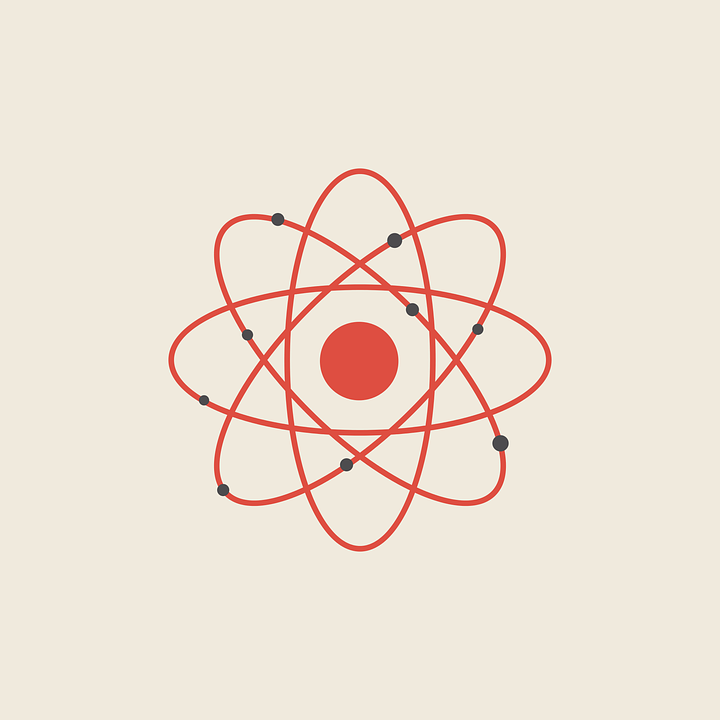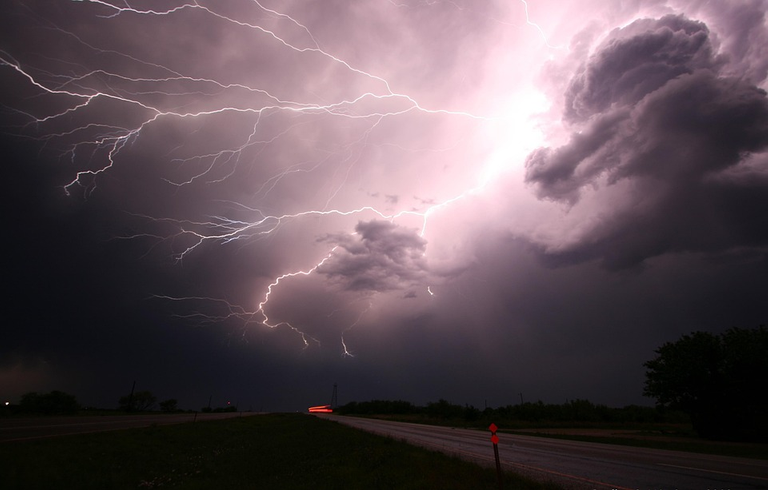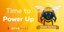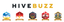# principles of physics

in StemSocial2 months agoSource

Basic physics refers to the branch of science that studies the nature and behavior of matter, energy, and their fundamental interactions. This science is based on the observation, analysis, interpretation and description of natural phenomena. This applies to both natural systems and artificial ones, such as robots and computers. This science is divided into several subdisciplines, each with its own areas of study.

### The fundamental principles of basic physics

The fundamental principles of basic physics lay the foundations for understanding natural phenomena. These principles are derived from the laws and theorems of physics, which provide guidance for the behavior of matter and energy. These principles are:

• The law of conservation of energy, which states that the total amount of energy in a closed system does not change with time.
• The law of conservation of momentum, which states that the total amount of momentum in a closed system does not change with time.
• The law of conservation of angular momentum, which states that the total angular momentum in a closed system does not change with time.
• The law of conservation of the amount of matter, which states that the total amount of matter in a closed system does not change with time.
• The law of conservation of the amount of energy, which states that the total amount of energy in a closed system does not change with time. This law applies both to systems in which energy is a constant quantity and to systems in which energy changes with time.Source

These principles and laws are used to study the effects of gravity, electromagnetism, kinetic energy, and potential energy. This allows physicists to understand natural phenomena and predict how bodies will behave when interacting with energy and matter.

### The branches of basic physics

The main branches of basic physics are classical mechanics, quantum mechanics, the theory of relativity, and statistical mechanics. These branches are studied from a mathematical perspective to understand the basic principles of physics.Source

• Classical mechanics: refers to the branch of physics that deals with the study of movements and mechanical phenomena. This branch is derived from Isaac Newton's laws of classical mechanics. These laws describe the relationship between force, momentum, and energy.
• Quantum mechanics: is the branch of physics that studies the behavior of atoms and light. This branch is based on the theory of quantum mechanics, which is a mathematical theory that models the behavior of matter and energy at the quantum level. This theory describes how energy behaves on a subatomic scale, and helps explain phenomena such as tunneling, virtual particles, and Hawking radiation.
• Theory of relativity: The theory of relativity is a theory of physics that describes gravity as a form of the curvature of space. This theory was proposed by Albert Einstein and is based on the idea that gravity is an effect of the curvature of space-time. This theory has been used to explain phenomena such as the force of gravity, black holes, and the displacement of light.
• Statistical Mechanics: Statistical mechanics is a branch of physics that studies the behavior of matter and energy in systems with many particles. This branch is based on the law of thermodynamics, which establishes the relationships between energy, temperature and other thermodynamic parameters. This theory is used to model the macroscopic behaviors of materials such as fluids, crystals, and gases.

## Bibliography

Thanks, @Alejos7ven.

Sort:Thanks for your contribution to the STEMsocial community. Feel free to join us on discord to get to know the rest of us!

Please consider delegating to the @stemsocial account (85% of the curation rewards are returned).

Thanks for including @stemsocial as a beneficiary, which gives you stronger support.

Congratulations @alejos7ven! You have completed the following achievement on the Hive blockchain And have been rewarded with New badge(s)You received more than 800 upvotes.Your next target is to reach 900 upvotes.

If you no longer want to receive notifications, reply to this comment with the word `STOP`Hive Power Up Day - February 1st 2023The Hive Gamification Proposal